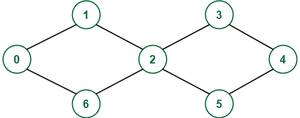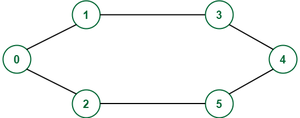Open in App
Not now

# Check if a cycle exists between nodes S and T in an Undirected Graph with only S and T repeating

• Difficulty Level : Easy
• Last Updated : 02 Feb, 2023

Given an undirected graph with N nodes and two vertices S & T, the task is to check if a cycle between these two vertices exists or not, such that no other node except S and T appear more than once in that cycle. Print Yes if it exists otherwise print No.

Example:

Input: N = 7, edges[][] = {{0, 1}, {1, 2}, {2, 3}, {3, 4}, {4, 5}, {5, 2}, {2, 6}, {6, 0}}, S = 0, T = 4
Output: No
Explanation:Node 2 appears two times, in the only cycle that exists between 0 & 4

Input: N = 6,  edges[][] = {{0, 1}, {0, 2}, {2, 5}, {3, 1}, {4, 5}, {4, 3}}, S = 0, T = 3
Output: Yes
Explanation:Cycle between 0 and 3 is: 0->1->3->4->5->2->0

Approach:

If there exists a path back from T to S that doesn’t have any vertices of the path used to travel to T from S, then there will always be a cycle such that no other node except S and T appears more than once. Now, to solve the problem follow the steps below:

1. Make an array visited of size n (where n is the number of nodes), and initialise it with all 0.
2. Start a depth-first search from S, and put T as the destination.
3. Change the value 0 of the current node to 1, in the visited array to keep the track of nodes visited in this path.
4. If it is not possible to reach T, then there is no way a simple cycle could exist between them. So, print No.
5. If T is reached, then change the destination to S and continue the depth-first search. Now, the nodes already visited can’t be visited again except S.
6. If S is reached then print Yes, otherwise No.

Below is the implementation of the above approach:

## C++

 `// C++ program for the above approach` `#include ``using` `namespace` `std;` `// Function to create graph``void` `createGraph(vector >& graph,``                 ``vector >& edges)``{``    ``for` `(``auto` `x : edges) {` `        ``// As it is an undirected graph``        ``// so add an edge for both directions``        ``graph[x].push_back(x);``        ``graph[x].push_back(x);``    ``}``}` `bool` `findSimpleCycle(``int` `cur,``                     ``vector >& graph,``                     ``int` `start, ``int` `dest,``                     ``vector<``bool``>& visited,``                     ``bool` `flag)``{` `    ``// After reaching T, change the dest to S``    ``if` `(!flag and cur == dest) {``        ``dest = start;``        ``flag = 1;``    ``}` `    ``// If S is reached without touching a``    ``// node twice except S and T,``    ``// then return true``    ``if` `(flag and cur == dest) {``        ``return` `true``;``    ``}` `    ``bool` `ans = 0;``    ``visited[cur] = 1;``    ``for` `(``auto` `child : graph[cur]) {` `        ``// A node can only be visited if it``        ``// hasn't been visited or if it``        ``// is S or t``        ``if` `(!visited[child] or child == dest) {``            ``ans = ans``                  ``| findSimpleCycle(``                        ``child, graph, start,``                        ``dest, visited, flag);``        ``}``    ``}` `    ``// Change visited of the current node``    ``// to 0 while backtracking again so``    ``// that all the paths can be traversed``    ``visited[cur] = 0;` `    ``return` `ans;``}` `int` `main()``{``    ``int` `nodes = 7;``    ``vector > edges``        ``= { { 0, 1 }, { 1, 2 }, { 2, 3 },``            ``{ 3, 4 }, { 4, 5 }, { 5, 2 },``            ``{ 2, 6 }, { 6, 0 } };``    ``int` `S = 0, T = 4;` `    ``// To store the graph``    ``vector > graph(nodes);` `    ``// To keep track of visited nodes``    ``vector<``bool``> visited(nodes);``    ``createGraph(graph, edges);` `    ``// If there exists a simple``    ``// cycle between S & T``    ``if` `(findSimpleCycle(S, graph,``                        ``S, T, visited, 0)) {``        ``cout << ``"Yes"``;``    ``}` `    ``// If no simple cycle exists``    ``// between S & T``    ``else` `{``        ``cout << ``"No"``;``    ``}``}`

## Java

 `import` `java.util.*;` `class` `Graph {``    ``// Function to create graph``    ``static` `void` `createGraph(List> graph,``                            ``List> edges) {``        ``for` `(List x : edges) {``            ``// As it is an undirected graph``            ``// so add an edge for both directions``            ``graph.get(x.get(``0``)).add(x.get(``1``));``            ``graph.get(x.get(``1``)).add(x.get(``0``));``        ``}``    ``}` `    ``static` `boolean` `findSimpleCycle(``int` `cur, List> graph,``                                ``int` `start, ``int` `dest, ``boolean``[] visited,``                                                     ``boolean` `flag) {` `        ``// After reaching T, change the dest to S``        ``if` `(!flag && cur == dest) {``            ``dest = start;``            ``flag = ``true``;``        ``}` `        ``// If S is reached without touching a``        ``// node twice except S and T,``        ``// then return true``        ``if` `(flag && cur == dest) {``            ``return` `true``;``        ``}` `        ``boolean` `ans = ``false``;``        ``visited[cur] = ``true``;``        ``for` `(``int` `child : graph.get(cur)) {` `            ``// A node can only be visited if it``            ``// hasn't been visited or if it``            ``// is S or t``            ``if` `(!visited[child] || child == dest) {``                ``ans = ans``                      ``| findSimpleCycle(child, graph, start, dest, visited, flag);``            ``}``        ``}` `        ``// Change visited of the current node``        ``// to 0 while backtracking again so``        ``// that all the paths can be traversed``        ``visited[cur] = ``false``;` `        ``return` `ans;``    ``}` `    ``public` `static` `void` `main(String[] args) {``        ``int` `nodes = ``7``;``        ``List> edges = Arrays.asList(``                ``Arrays.asList(``0``, ``1``),``                ``Arrays.asList(``1``, ``2``),``                ``Arrays.asList(``2``, ``3``),``                ``Arrays.asList(``3``, ``4``),``                ``Arrays.asList(``4``, ``5``),``                ``Arrays.asList(``5``, ``2``),``                ``Arrays.asList(``2``, ``6``),``                ``Arrays.asList(``6``, ``0``)``        ``);``        ``int` `S = ``0``, T = ``4``;` `        ``// To store the graph``        ``List> graph = ``new` `ArrayList<>();``        ``for` `(``int` `i = ``0``; i < nodes; i++) {``            ``graph.add(``new` `ArrayList());``        ``}` `        ``// To keep track of visited nodes``        ``boolean``[] visited = ``new` `boolean``[nodes];``        ``createGraph(graph, edges);` `        ``// If there exists a simple``        ``// cycle between S & T``        ``if` `(findSimpleCycle(S, graph, S, T, visited, ``false``)) {``            ``System.out.println(``"Yes"``);``        ``}` `        ``// If no simple cycle exists``        ``// between S & T``        ``else` `{``            ``System.out.println(``"No"``);``        ``}``    ``}``}``// this code is contributed by devendrasalunke`

## Python3

 `# Function to create graph``def` `createGraph(edges, N):``    ``graph ``=` `list``([] ``for` `_ ``in` `range``(N))``    ``for` `node1, node2 ``in` `edges:` `        ``# As it is an undirected graph,``        ``# add an edge for both directions``        ``graph[node1].append(node2)``        ``graph[node2].append(node1)` `    ``return` `graph`  `def` `findSimpleCycle(cur,``                    ``graph,``                    ``start,  dest,``                    ``visited,``                    ``flag):` `    ``# After reaching T, change the dest to S``    ``if` `((``not` `flag) ``and` `cur ``=``=` `dest):``        ``dest ``=` `start``        ``flag ``=` `True` `    ``# If S is reached without touching a``    ``# node twice except S and T,``    ``# then return true``    ``if` `(``not` `flag ``and` `cur ``=``=` `dest):``        ``return` `True` `    ``# first guess is that there is no cycle``    ``# so ans is False.``    ``# if we find one cycle, ans will be true``    ``# and then returned .``    ``ans ``=` `False` `    ``# mark node as visited in this path``    ``visited[cur] ``=` `True` `    ``for` `child ``in` `graph[cur]:` `        ``# A node can only be visited if it``        ``# hasn't been visited or if it``        ``# is S or t``        ``if` `(``not` `visited[child]) ``or` `child ``=``=` `dest:``            ``ans ``=` `ans ``or` `findSimpleCycle(``                ``child, graph, start,``                ``dest, visited, flag)` `    ``# Change visited of the current node``    ``# to 0 while backtracking again so``    ``# that all the paths can be traversed``    ``visited[cur] ``=` `False` `    ``return` `ans`  `if` `__name__ ``=``=` `"__main__"``:` `    ``N ``=` `7`  `# number of nodes``    ``edges ``=` `[[``0``, ``1``], [``1``, ``2``], [``2``, ``3``],``             ``[``3``, ``4``], [``4``, ``5``], [``5``, ``2``],``             ``[``2``, ``6``], [``6``, ``0``]]``    ``S ``=` `0``    ``T ``=` `4` `    ``# To keep track of visited nodes``    ``visited_array ``=` `list``(``False` `for` `_ ``in` `range``(N))` `    ``# If there exists a simple``    ``# cycle between S & T``    ``if` `(findSimpleCycle(cur``=``S, graph``=``createGraph(edges, N),``                        ``start``=``S, dest``=``T,``                        ``visited``=``visited_array,``                        ``flag``=``0``)):``        ``print``(``"Yes"``)` `    ``# If no simple cycle exists``    ``# between S & T``    ``else``:``        ``print``(``"No"``)`

## C#

 `using` `System;``using` `System.Collections.Generic;` `class` `Graph {``    ``// Function to create graph``    ``static` `void` `createGraph(List > graph,``                            ``List > edges)``    ``{``        ``foreach``(List<``int``> x ``in` `edges)``        ``{``            ``// As it is an undirected graph``            ``// so add an edge for both directions``            ``graph[x].Add(x);``            ``graph[x].Add(x);``        ``}``    ``}` `    ``static` `bool` `findSimpleCycle(``int` `cur,``                                ``List > graph,``                                ``int` `start, ``int` `dest,``                                ``bool``[] visited, ``bool` `flag)``    ``{` `        ``// After reaching T, change the dest to S``        ``if` `(!flag && cur == dest) {``            ``dest = start;``            ``flag = ``true``;``        ``}` `        ``// If S is reached without touching a``        ``// node twice except S and T,``        ``// then return true``        ``if` `(flag && cur == dest) {``            ``return` `true``;``        ``}` `        ``bool` `ans = ``false``;``        ``visited[cur] = ``true``;``        ``foreach``(``int` `child ``in` `graph[cur])``        ``{` `            ``// A node can only be visited if it``            ``// hasn't been visited or if it``            ``// is S or t``            ``if` `(!visited[child] || child == dest) {``                ``ans = ans``                      ``| findSimpleCycle(child, graph, start,``                                        ``dest, visited,``                                        ``flag);``            ``}``        ``}` `        ``// Change visited of the current node``        ``// to 0 while backtracking again so``        ``// that all the paths can be traversed``        ``visited[cur] = ``false``;` `        ``return` `ans;``    ``}` `    ``public` `static` `void` `Main(``string``[] args)``    ``{``        ``int` `nodes = 7;``        ``List > edges = ``new` `List >() {``            ``new` `List<``int``>() { 0, 1 },``                ``new` `List<``int``>() { 1, 2 },``                ``new` `List<``int``>() { 2, 3 },``                ``new` `List<``int``>() { 3, 4 },``                ``new` `List<``int``>() { 4, 5 },``                ``new` `List<``int``>() { 5, 2 },``                ``new` `List<``int``>() { 2, 6 },``                ``new` `List<``int``>() { 6, 0 }``        ``};``        ``int` `S = 0, T = 4;` `        ``// To store the graph``        ``List > graph = ``new` `List >();``        ``for` `(``int` `i = 0; i < nodes; i++) {``            ``graph.Add(``new` `List<``int``>());``        ``}` `        ``// To keep track of visited nodes``        ``bool``[] visited = ``new` `bool``[nodes];``        ``createGraph(graph, edges);` `        ``// If there exists a simple``        ``// cycle between S & T``        ``if` `(findSimpleCycle(S, graph, S, T, visited,``                            ``false``)) {``            ``Console.WriteLine(``"Yes"``);``        ``}` `        ``// If no simple cycle exists``        ``// between S & T``        ``else` `{``            ``Console.WriteLine(``"No"``);``        ``}``    ``}``}`

## Javascript

 ``

Output

`No`

Time Complexity: O(N!).   As we can see in this algorithm, all paths can be traversed, in the worst case, we are going to traverse all paths to find one that works, see this article: https://www.geeksforgeeks.org/count-possible-paths-two-vertices/
Auxiliary Space: O(N^2)

My Personal Notes arrow_drop_up